# Difference between revisions of "Supercooling and nucleation"

Supercooling, undercooling and nucleation.

## Volmer and Weber kinetic model

Volmer and Weber kinetic model  results in the following nucleation rate: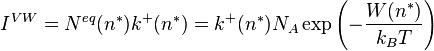$I^{VW} = N^{eq}(n^*) k^+(n^*) = k^+(n^*) N_A \exp \left( -\frac{W(n^*)}{k_BT} \right)$

## Homogeneous nucleation temperature

The homogeneous nucleation temperature (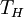$T_H$) is the temperature below which it is almost impossible to avoid spontaneous and rapid freezing.

## Zeldovich factor

The Zeldovich factor  (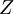$Z$) modifies the Volmer and Weber expression, making it applicable to spherical clusters: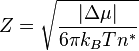$Z= \sqrt{\frac{ \vert \Delta \mu \vert }{6 \pi k_B T n^*}}$

## Zeldovich-Frenkel equation

Zeldovich-Frenkel master equation is given by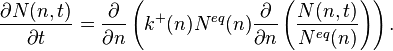$\frac{\partial N(n, t)}{\partial t} = \frac{\partial }{\partial n} \left( k^+ (n) N^{eq} (n) \frac{\partial }{\partial n} \left( \frac{N(n, t)}{N^{eq}(n)} \right) \right).$